Ten Minute Physics

This is the page accompanying my youtube channel Ten Minute Physics. In short episodes of about ten minutes I explain the basic concepts of physically based simulation. Each time I write a little javascript demo that runs in any browswer.

## Tutorials

Many more to come - stay tuned!18 How to write a FLIP Water Simulator. In this tutorial I explain the FLIP method. It is an extension of the Eulerian fluid simulation method which uses particles to distinguish air from water cells. Video Code Demo Notes (PDF)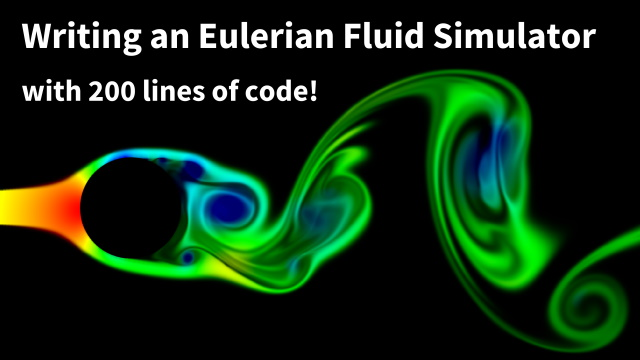17 How to write an Eulerian Fluid Simulator with 200 lines of code. In this tutorial I explain the basics of Eulerian, grid-based fluid simulation and show how to write a simulation engine based on these discussed concepts. Video Code Demo Notes (PDF)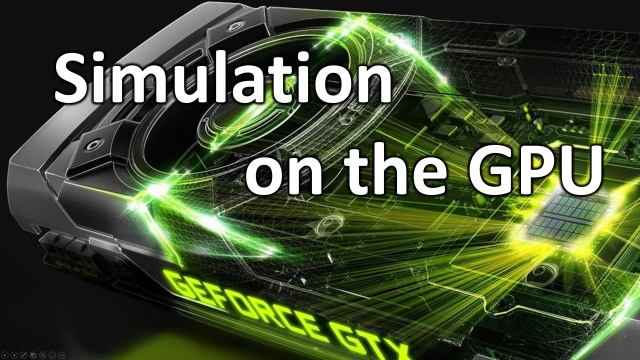16 Simulation on the GPU In this tutorial I give a short introduction to simulating on the GPU. I use python in connection with the nvidia python extension warp as a simple way to write GPU simulations. Video Code Notes (PDF)15 Self-collisions, solving the hardest problem in animation In this tutorial I give you 5 tricks for stably handling cloth self-collisions. We use the cloth simulation of tutorial 14 and the hash grid introduced in tutorial 11. Video Code Demo Notes (PDF)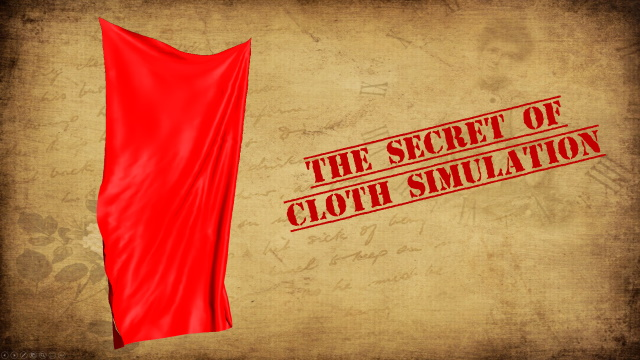14 The secret of cloth simulation In this tutorial I reveal the secret of cloth simulation. I use it to write a very fast and stable cloth demo that simulates 6400 triangles at more than 30 fps on a cell phone in javascript. Video Code Demo Notes (PDF)13 Writing a Tetrahedralizer for Blender In this tutorial I explain how to create a tetrahedral mesh for a surface mesh using the incremental Delaunay method. I also briefly discuss the implementation as a python blender plugin. Video Plugin Notes (PDF)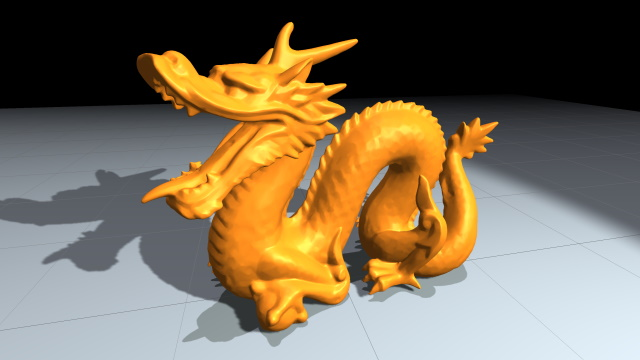12 100x speedup for soft body simulations In this tutorial I show how to speedup soft body simulation by 100x by embedding a high detail visual mesh into a low resolution tetrahedral simulation mesh. Video Code Demo Notes (PDF)11 Finding overlaps among thousands of objects blazing fast In this tutorial I show how to find overlaps among thousands of objects using spatial hashing. In addition to explaining the method, I also show how to implement it in a very efficient way. Video Code Demo Notes (PDF)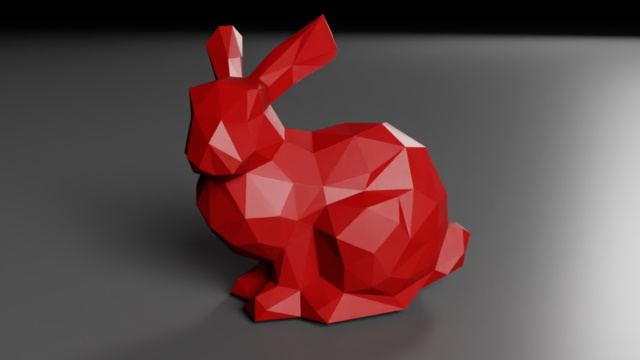10 Simple and unbreakable simulation of soft bodies In this tutorial I show how to simulate soft bodies with in an unconditionally stable and simple way. Using a discrete rather than a continuous model and a local instead of a global solver removes almost all difficulties of traditional methods and yields a simple, short and fast algorithm. I recommend to watch tutorial 9 first. Video Code Demo Notes (PDF)09 Getting ready to simulate the world with XPBD In this tutorial I introduce general extended position based dynamics or XPBD, a simple and unbreakable method to simulate almost everything. The tutorial is self contained. However, I highly recommend to watch tutorial 7 first to refresh your 3d math. Video Notes (PDF)08 Providing user interaction with a 3d scene In this tutorial I show how to enable the user to pick up move and throw objects in a 3d scene. For this I introduce the concepts of a mouse ray and ray casting. Video Code Demo07 Intuitive 3d Vector Math for Simulation I give an introduction to 3d vector math, the math that we need to write 3d simulations. In the compact presentation I focus on the part used in simulations. In addition to the definitions of the concepts, I explain my personal intuitions behind the concepts which I developed over time. Video Notes (PDF)06 Writing a triple pendulum simulation is simple I show you how to handle hard distance constraints with Position based dynamics. They can be used to simulate a large variety of objects like cloth, ropes, hair, fur, sand and many more. To demonstrate the accuracy of PBD we will write a triple pendulum simulation. Video Short Code Short Demo       Code Demo05 The simplest possible physics simulation method I introduce the Position Based Dynamics method to simulate constraints. I apply it to the circle on wire problem and compare it to the analytic solution to demonstrate its accuracy. Video Code Demo       Code Demo04 How to write a pinball simulation I show you how to handle ball - capsule collisions and collisions against arbitrary boundaries represented by segments. I also show how to handle touch and mouse events. Video Code Demo03 - Ball collision handling in 2d I show how to handle collisions of balls in 2d while creating a billiard scene. Video Code Demo02 - Introduction to 3d and VR web browser physics We make our cannonball 3d and turn it into a VR demo using the graphics engine THREE.js. Video Code Demo       VR Code VR Demo01 - Introduction to 2d web browser physics I give a brief intoduction to this channel and to physics. After that we write a 2d cannonball simulation. Video Code Demo